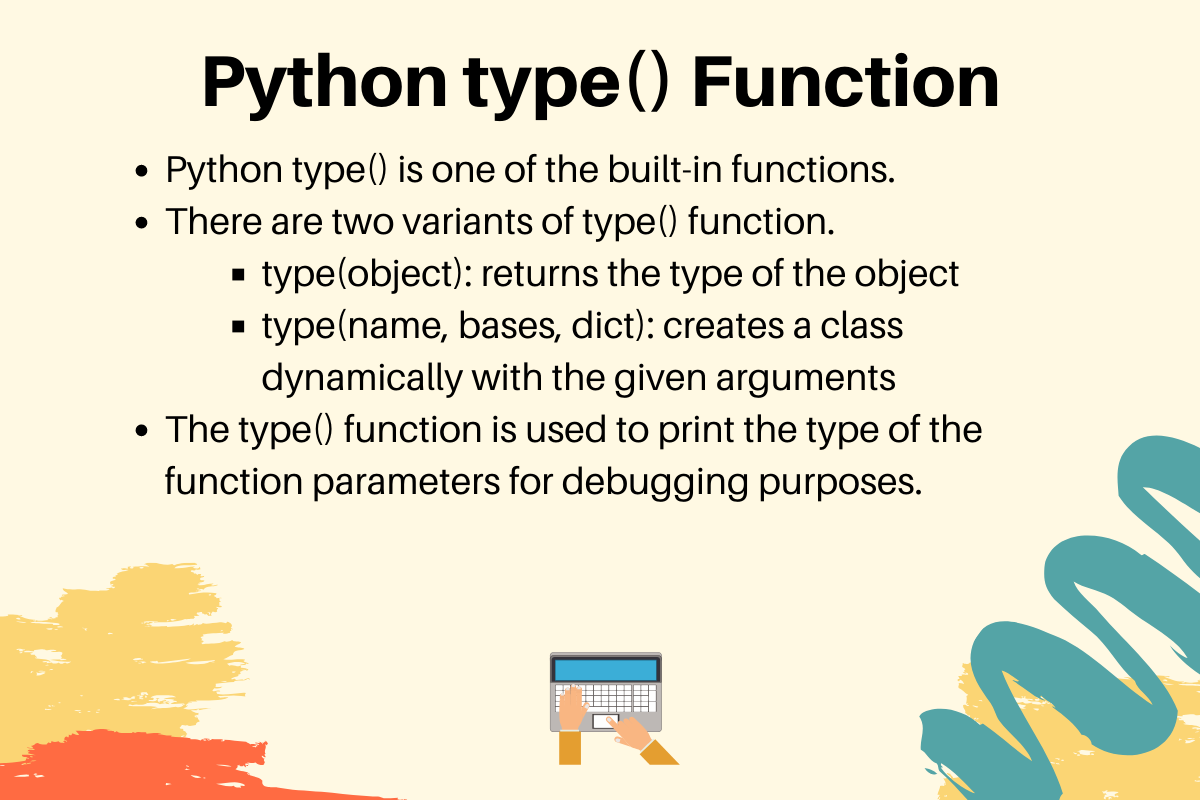# Python type() Function [With Easy Examples]

Published on August 3, 2022By PankajWhile we believe that this content benefits our community, we have not yet thoroughly reviewed it. If you have any suggestions for improvements, please let us know by clicking the “report an issue“ button at the bottom of the tutorial.

We use the type() function in Python to identify the type of a specific Python object. It’s a very straightforward function and an easy to understand one for that. Without any further ado, let’s get right into the syntax.

## Syntax of the Python type() function

Python has a lot of built-in functions. The `type()` function is used to get the type of an object.

Python type() function syntax is:

``````type(object)

type(name, bases, dict)
``````

When a single argument is passed to the type() function, it returns the type of the object. Its value is the same as the object.__class__ instance variable.

When three arguments are passed, it returns a new type object. It’s used to create a class dynamically on the fly.

• “name” string becomes the class name. It’s the same as the __name__ attribute of a class.
• “bases” tuple specifies the base classes. It’s the same as the __bases__ attribute of the class.
• “dict” dictionary helps create the class body. It’s the same as the __dict__ attribute of the class.

## Examples of the type() function in Python

Let’s look into some examples of using the type() function.

### 1. Finding the type of a Python object

``````x = 10
print(type(x))

s = 'abc'
print(type(s))

from collections import OrderedDict

od = OrderedDict()
print(type(od))

class Data:
pass

d = Data()
print(type(d))
``````

Output:

``````<class 'int'>
<class 'str'>
<class 'collections.OrderedDict'>
<class '__main__.Data'>
``````

Notice that the type() function returns the type of the object with the module name. Since our Python script doesn’t have a module, it’s module becomes __main__.

### 2. Extracting Details from Python Classes

Let’s say we have following classes. We’ll pull metadata about the classes using the class, bases, dict, and doc properties.

``````class Data:
"""Data Class"""
d_id = 10

class SubData(Data):
"""SubData Class"""
sd_id = 20

``````

Let’s print some of the properties of these classes.

``````print(Data.__class__)
print(Data.__bases__)
print(Data.__dict__)
print(Data.__doc__)

print(SubData.__class__)
print(SubData.__bases__)
print(SubData.__dict__)
print(SubData.__doc__)

``````

Output:

``````<class 'type'>
(<class 'object'>,)
{'__module__': '__main__', '__doc__': 'Data Class', 'd_id': 10, '__dict__': <attribute '__dict__' of 'Data' objects>, '__weakref__': <attribute '__weakref__' of 'Data' objects>}
Data Class

<class 'type'>
(<class '__main__.Data'>,)
{'__module__': '__main__', '__doc__': 'SubData Class', 'sd_id': 20}
SubData Class
``````

We can create similar classes using the type() function.

``````Data1 = type('Data1', (object,), {'__doc__': 'Data1 Class', 'd_id': 10})
SubData1 = type('SubData1', (Data1,), {'__doc__': 'SubData1 Class', 'sd_id': 20})

print(Data1.__class__)
print(Data1.__bases__)
print(Data1.__dict__)
print(Data1.__doc__)

print(SubData1.__class__)
print(SubData1.__bases__)
print(SubData1.__dict__)
print(SubData1.__doc__)

``````

Output:

``````<class 'type'>
(<class 'object'>,)
{'__doc__': 'Data1 Class', 'd_id': 10, '__module__': '__main__', '__dict__': <attribute '__dict__' of 'Data1' objects>, '__weakref__': <attribute '__weakref__' of 'Data1' objects>}
Data1 Class

<class 'type'>
(<class '__main__.Data1'>,)
{'__doc__': 'SubData1 Class', 'sd_id': 20, '__module__': '__main__'}
SubData1 Class

``````

Note that we can’t create functions in the dynamic class using the type() function.

## Real-Life Usage of the type() function

Python is a dynamically-typed language. So, if we want to know the type of the arguments, we can use the type() function. If you want to make sure that your function works only on the specific types of objects, use isinstance() function.

Let’s say we want to create a function to calculate something on two integers. We can implement it in the following way.

``````def calculate(x, y, op='sum'):
if not(isinstance(x, int) and isinstance(y, int)):
print(f'Invalid Types of Arguments - x:{type(x)}, y:{type(y)}')
raise TypeError('Incompatible types of arguments, must be integers')

if op == 'difference':
return x - y
if op == 'multiply':
return x * y
# default is sum
return x + y

``````

The isinstance() function is used to validate the input argument type. The type() function is used to print the type of the parameters when validation fails.

Click below to sign up and get \$200 of credit to try our products over 60 days!

### Popular Topics

##### Join the Tech Talk##### Hollie's Hub for Good

Working on improving health and education, reducing inequality, and spurring economic growth? We’d like to help.